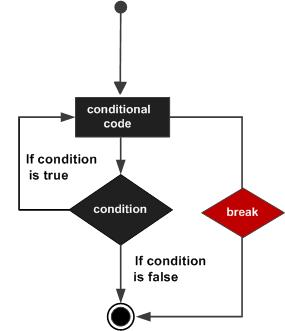# Pascal - Break Statement

#### Learn Pascal Programming from Scratch

94 Lectures 8.5 hours

The break statement in Pascal has the following two usages −

• When the break statement is encountered inside a loop, the loop is immediately terminated and program control resumes at the next statement following the loop.

• It can be used to terminate a case in the case statement (covered in the next chapter).

If you are using nested loops (i.e., one loop inside another loop), the break statement will stop the execution of the innermost loop and start executing the next line of code after the block.

## Syntax

The syntax for a break statement in Pascal is as follows −

```break;
```

## Flow Diagram### Example

```program exBreak;
var
a: integer;

begin
a := 10;
(* while loop execution *)
while  a < 20 do

begin
writeln('value of a: ', a);
a:=a +1;

if( a > 15) then
(* terminate the loop using break statement *)
break;
end;
end.
```

When the above code is compiled and executed, it produces the following result −

```value of a: 10
value of a: 11
value of a: 12
value of a: 13
value of a: 14
value of a: 15
```
pascal_loops.htm